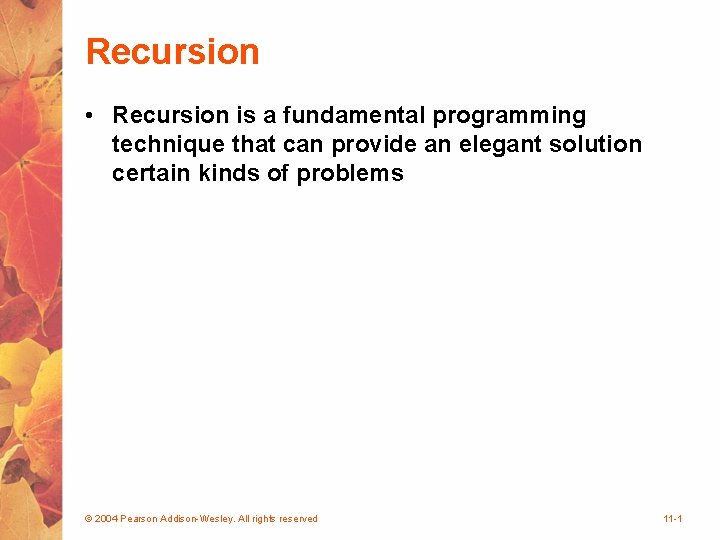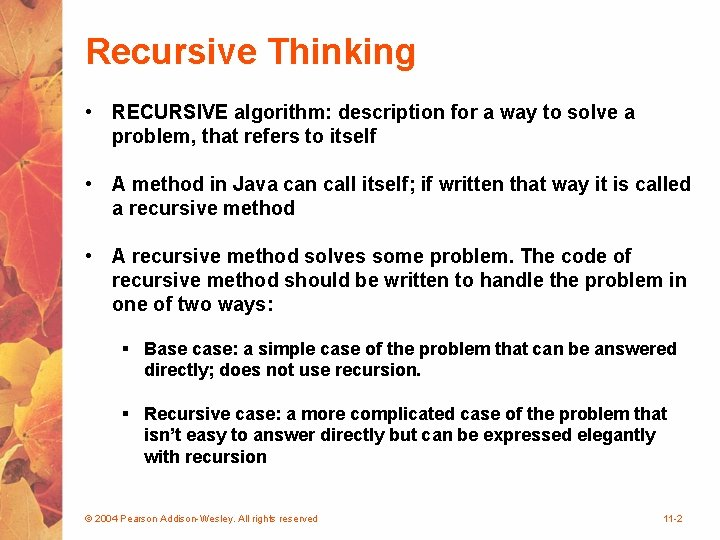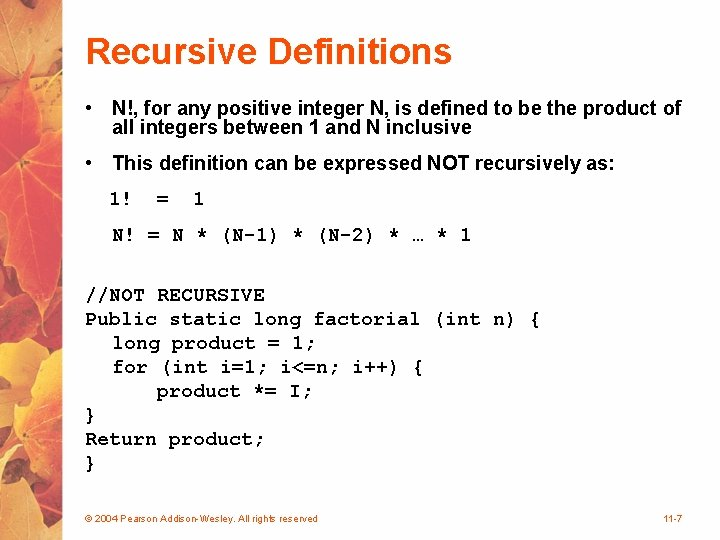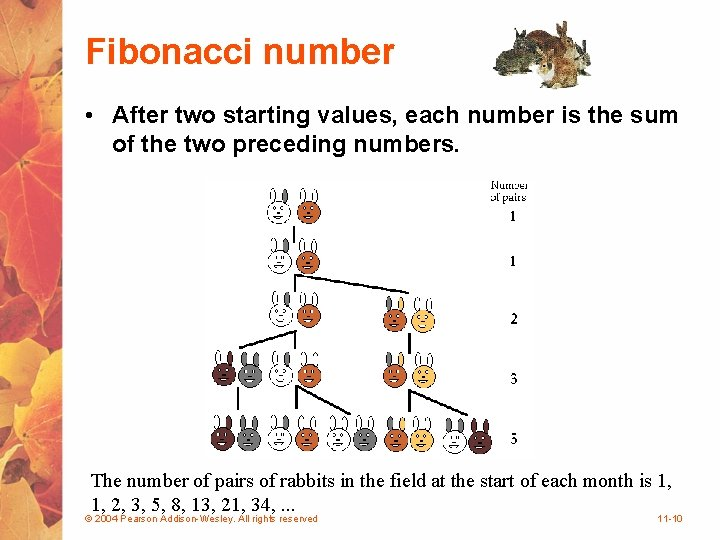# Recursion Recursion is a fundamental programming technique that

• Slides: 10Recursive Thinking • RECURSIVE algorithm: description for a way to solve a problem, that refers to itself • A method in Java can call itself; if written that way it is called a recursive method • A recursive method solves some problem. The code of recursive method should be written to handle the problem in one of two ways: § Base case: a simple case of the problem that can be answered directly; does not use recursion. § Recursive case: a more complicated case of the problem that isn’t easy to answer directly but can be expressed elegantly with recursion © 2004 Pearson Addison-Wesley. All rights reserved 11 -2Recursive Definitions • Consider the following list of numbers: 24, 88, 40, 37 • Such a list can be defined as follows: A LIST is a: or a: number comma LIST • That is, a LIST is defined to be a single number, or a number followed by a comma followed by a LIST • The concept of a LIST is used to define itself © 2004 Pearson Addison-Wesley. All rights reserved 11 -3Recursive Definitions • The recursive part of the LIST definition is used several times, terminating with the non-recursive part: number comma LIST 24 , 88, 40, 37 number comma LIST 88 , 40, 37 number comma LIST 40 , 37 number 37 © 2004 Pearson Addison-Wesley. All rights reserved 11 -4Recursive definitions • List of numbers: § 24 → 88→ 40→ 37/ • A list can be defined recursively: § LIST = null (EMPTY) § LIST = element → LIST © 2004 Pearson Addison-Wesley. All rights reserved 11 -5Infinite Recursion • All recursive definitions have to have a nonrecursive part • If they didn't, there would be no way to terminate the recursive path • Such a definition would cause infinite recursion • This problem is similar to an infinite loop, but the non-terminating "loop" is part of the definition itself • The non-recursive part is often called the base case © 2004 Pearson Addison-Wesley. All rights reserved 11 -6Recursive Definitions • N!, for any positive integer N, is defined to be the product of all integers between 1 and N inclusive • This definition can be expressed NOT recursively as: 1! = 1 N! = N * (N-1) * (N-2) * … * 1 //NOT RECURSIVE Public static long factorial (int n) { long product = 1; for (int i=1; i<=n; i++) { product *= I; } Return product; } © 2004 Pearson Addison-Wesley. All rights reserved 11 -7Recursive factorial Factorial can also be defined recursively: N! = N * (N-1)! • A factorial is defined in terms of another factorial • Eventually, the base case of 1! is reached //RECURSIVE Public static long factorial (int n) { if (n== 0) { return 1; } else { return n * factorial (n-1); }} © 2004 Pearson Addison-Wesley. All rights reserved 11 -8Recursive Definitions 5! 120 5 * 4! 24 4 * 3! 6 3 * 2! 2 * 1! 2 1 © 2004 Pearson Addison-Wesley. All rights reserved 11 -9Fibonacci number • After two starting values, each number is the sum of the two preceding numbers. The number of pairs of rabbits in the field at the start of each month is 1, 1, 2, 3, 5, 8, 13, 21, 34, . . . © 2004 Pearson Addison-Wesley. All rights reserved 11 -10